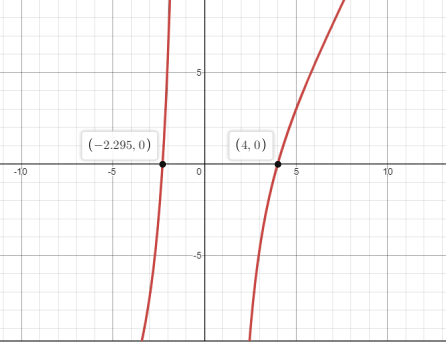# Tofind: the point on hyperbola which is closest to the point given below.### Single Variable Calculus: Concepts...

4th Edition
James Stewart
Publisher: Cengage Learning
ISBN: 9781337687805### Single Variable Calculus: Concepts...

4th Edition
James Stewart
Publisher: Cengage Learning
ISBN: 9781337687805

#### Solutions

Chapter 4, Problem 40RE
To determine

## Tofind:the point on hyperbola which is closest to the point given below.

Expert Solution

Thepoint which is closest to (3,0) is (4,84) or (4,2)

### Explanation of Solution

Given:

xy=8 .

Closest point is (3,0) .

Concept used:

Distance formula:

d=(xx1)2+(yy1)2 .

Local minima Occurs when f(x) changes its sign from negative to positive.

Calculation:

Every point on the hyperbola xy=8 is in the form of (x,8x) .

Distance between the point (x,8x) and (3,0) is

d(x)=(x-3)2+(8x-0)2 .

Her to find the x foe which the d(x) id minimum.

That is

f(x)=x2-6x+9+64x2 .

f(x)=2x-6-128x3

The graph of the f(x) is shown below:Local minima Occurs when f(x) changes its sign from negative to positive.

Therefore, the graph above,shows that the local minima occurs when x=4 .

Since there is only one local Minima and limx±f(x)= .

Hence the point which is closest to (3,0) is (4,84) or (4,2) .

### Have a homework question?

Subscribe to bartleby learn! Ask subject matter experts 30 homework questions each month. Plus, you’ll have access to millions of step-by-step textbook answers!